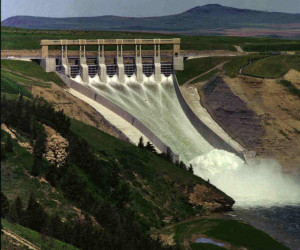# How to Use the Bernoulli EquationThe Bernoulli equation states that the total energy possessed by a fluid is the sum of its pressure, kinetic, and potential energies.$E_t = E_p + E_v + E_z\newline \indent =\frac{p}{\rho}+\frac{v^{2}}{2}+zg\hspace{20px}^{(SI)}\newline \indent =\frac{p}{\rho}+\frac{v^{2}}{2g_c}+\frac{zg}{g_c}\hspace{20px}^{(US)}$

Using this starting point, we can calculate the total head, ht, and total pressure, pt, that the fluid is under:$h_t = \frac{E_t}{g}\hspace{30px}^{(SI)}\newline h_t = E_t \times \frac{g_c}{g}\hspace{30px}^{(US)}\newline$$p_t = \rho g h_t\hspace{30px}^{(SI)}\newline p_t = \rho h_t \times \frac{g}{g_c}\hspace{30px}^{(US)}$

Calculating the energy of a liquid is purely a theoretical exercise, but the latter two formulas are used in design.

If there is no pressure involved, it reduces the problem to a fairly simple form. For instance, the water behind a dam is at rest, and its potential energy can easily be calculated. When it is released, the Bernoulli equation states that the potential energy turns into potential and kinetic energy, and the velocity of the flow can be calculated.

## Assumptions

The Bernoulli equations makes the following assumptions:

1. The fluid incompressible.
2. There is no friction involved in fluid flow.
3. Thermal energy changes are nil or negligible.

This site uses Akismet to reduce spam. Learn how your comment data is processed.